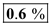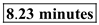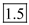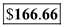# Quiz 2: The Process View of the Organization

Business

Inventory Cost is the Cost associated with Ordering, Holding, Obsolescence Pilferage cost of the Inventory which is expressed as a percentage of the Inventory Valuation Consider the following data given in the question: Inventory in D's 10-k = \$400 Cost of goods sold in D's 10-k = \$26,442 Calculate the Inventory Turns for company D for the year 2001 by using the formula as follows:Calculate the cost of inventory per unit from the formula as follows:Therefore, the percentage of cost a D computer reflects inventory costs isLittle's Law gives us a relationship between Average Inventory, Flow rate and the Flow Time. Arrival rate is the flow rate of the passengers. The average time the passenger spends in the system is the Flow Time. Consider the following data given in the question: Arrival rate or Flow rate = 255 Passenger per hour Average Inventory of passengers = 35 passengers Calculate the flow time, using Little's law, as follows:Therefore, the average passenger has to wait in line isInventory Turns is the inverse of the Flow time. Cost of the Goods Sold is the Cost of the production that company incurs in making the goods when it is sold. Inventory turns is the Number of times the inventory is sold or used in a year or a period of time. Consider the following data given in the question: Cost of Goods Sold as \$1000 per unit for a Sales Cost of \$2000 per unit. Note: Since \$60,000,000 sales were realized in the last year, it can be concluded that the COGS for this value will be half of the sales. Calculate the Cost of Goods Sold from sales of \$60,000,000 as \$30,000,000 as follows:Therefore, the company turns its inventory inb) Calculate the cost of inventory per unit from the formula as follows:Therefore, for a product costing \$1000 the Inventory Cost would be 16.66% of \$1000 is